• 启动Python学习的第一个实例：一个正弦函数、余弦函数坐标系的实例解析

# -*- coding: UTF-8 -*-

# Imports
# 用 import 导入模块，安装“模块.函数”的格式使用这个模块的函数。
import numpy as np
import matplotlib.pyplot as plt

# Create a new figure of size 8x6 points, using 100 dots per inch
# 创建一个 8 * 6 点（point）的图，并设置分辨率为 100。
plt.figure(figsize=(10,6), dpi=80)

# Create a new subplot from a grid of 1x1
plt.subplot(111)

# linspace()和matlab的linspace很类似，用于创建指定数量等间隔的序列，实际生成一个等差数列；
# 生成首位是-π，末位是π，含256个数的等差数列。
X = np.linspace(-np.pi, np.pi, 256,endpoint=True)
C = np.cos(X)
S = np.sin(X)

# Plot cosine using blue color with a continuous line of width 1 (pixels)
plt.plot(X, C, color="blue", linewidth=2.0, linestyle="-")

# Plot sine using green color with a continuous line of width 1 (pixels)
plt.plot(X, S, color="red", linewidth=2.0, linestyle="-")

# Set x limits
# plt.xlim(-4.0,4.0)
# 图片边界设置得不好，所以有些地方看得不是很清楚。因此进行调整。
plt.xlim(X.min()*1.1, X.max()*1.1)

# Set x ticks
# plt.xticks(np.linspace(-4,4,9,endpoint=True))
# 我们讨论正弦和余弦函数的时候，通常希望知道函数在“±π”和“±π/2”的值。因此进行如下调整
# plt.xticks( [-np.pi, -np.pi/2, 0, np.pi/2, np.pi])
plt.xticks([-np.pi, -np.pi/2, 0, np.pi/2, np.pi],
[r'$-\pi$', r'$-\pi/2$', r'$0$', r'$+\pi/2$', r'$+\pi$'])

# Set y limits
# plt.ylim(-1.0,1.0)
# 图片边界设置得不好，所以有些地方看得不是很清楚。因此进行调整。
plt.ylim(C.min()*1.1, C.max()*1.1)

# Set y ticks
plt.yticks(np.linspace(-1,1,5,endpoint=True))
# plt.yticks([-1, 0, +1])

# 坐标轴线和上面的记号连在一起就形成了脊柱（Spines，一条线段上有一系列的凸起，是不是很像脊柱骨啊~），
# 它记录了数据区域的范围。它们可以放在任意位置，不过至今为止，我们都把它放在图的四边。
# 实际上每幅图有四条脊柱（上下左右），为了将脊柱放在图的中间，
# 我们必须将其中的两条（上和右）设置为无色，然后调整剩下的两条到合适的位置——数据空间的 0 点。

ax = plt.gca()
ax.spines['right'].set_color('none')
ax.spines['top'].set_color('none')
ax.xaxis.set_ticks_position('bottom')
ax.spines['bottom'].set_position(('data',0))
ax.yaxis.set_ticks_position('left')
ax.spines['left'].set_position(('data',0))

# 我们在图的左上角添加一个图例。为此，我们只需要在 plot 函数里以「键 - 值」的形式增加一个参数。
plt.plot(X, C, color="blue", linewidth=2.5, linestyle="-", label="cos")
plt.plot(X, S, color="red",  linewidth=2.5, linestyle="-", label="sin")

# plt.legend(loc='upper left', frameon=False)
# bbox_to_anchor被赋予的二元组中，第一个数值用于控制legend的左右移动，值越大越向右边移动，
# 第二个数值用于控制legend的上下移动，值越大，越向上移动。
# plt.plot的label文字大小在plt.legend中设置：fontsize

# 我们希望在“2π/3”的位置给两条函数曲线加上一个注释。
# 首先，我们在对应的函数图像位置上画一个点；
# 然后，向横轴引一条垂线，以虚线标记；最后，写上标签。
# cos
t = 2*np.pi/3
plt.plot([t,t],[0,np.cos(t)], color ='blue', linewidth=1.5, linestyle="--")
plt.scatter([t,],[np.cos(t),], 50, color ='blue')
plt.annotate(r'$\cos(\frac{2\pi}{3})=-\frac{1}{2}$',
xy=(t, np.cos(t)), xycoords='data',
xytext=(-90, -50), textcoords='offset points', fontsize=16,
# sin
plt.plot([t,t],[0,np.sin(t)], color ='red', linewidth=1.5, linestyle="--")
plt.scatter([t,],[np.sin(t),], 50, color ='red')
plt.annotate(r'$\sin(\frac{2\pi}{3})=\frac{\sqrt{3}}{2}$',
xy=(t, np.sin(t)), xycoords='data',
xytext=(+10, +30), textcoords='offset points', fontsize=16,

# 坐标轴上的记号标签被曲线挡住了，作为强迫症患者这是不能忍的。
# 我们可以把它们放大，然后添加一个白色的半透明底色。这样可以保证标签和曲线同时可见。
for label in ax.get_xticklabels() + ax.get_yticklabels():
label.set_fontsize(16)
label.set_bbox(dict(facecolor='white', edgecolor='None', alpha=0.65 ))

# Save figure using 72 dots per inch
# savefig("../figures/exercice_2.png",dpi=72)
# http://www.labri.fr/perso/nrougier/teaching/matplotlib/

# Show result on screen
plt.show()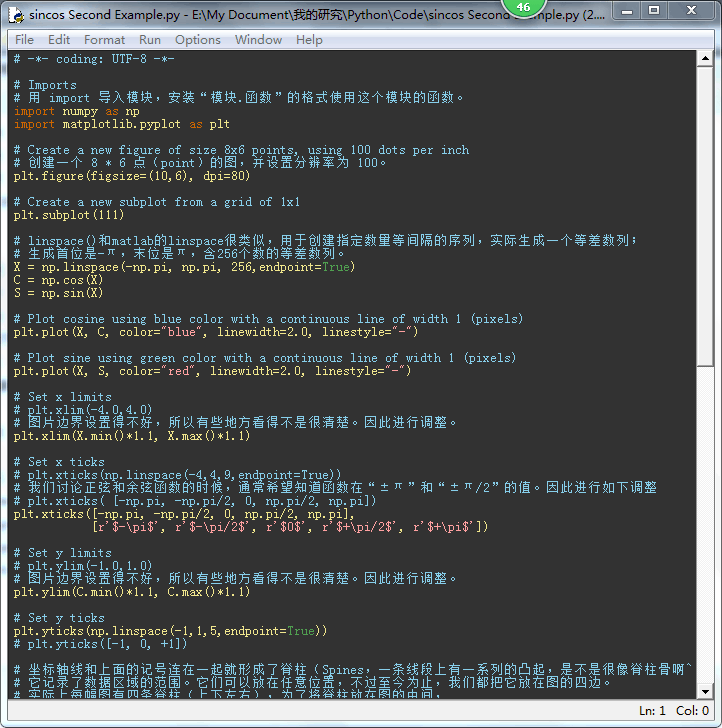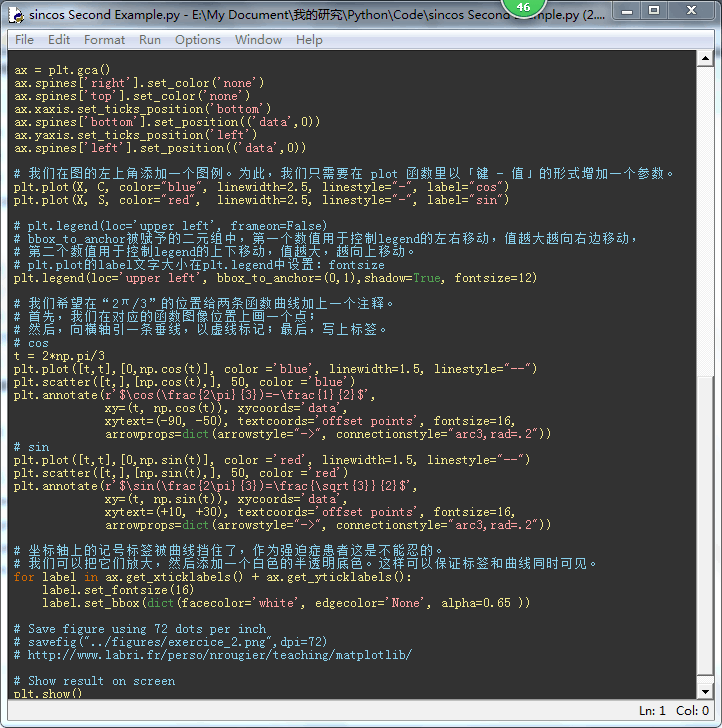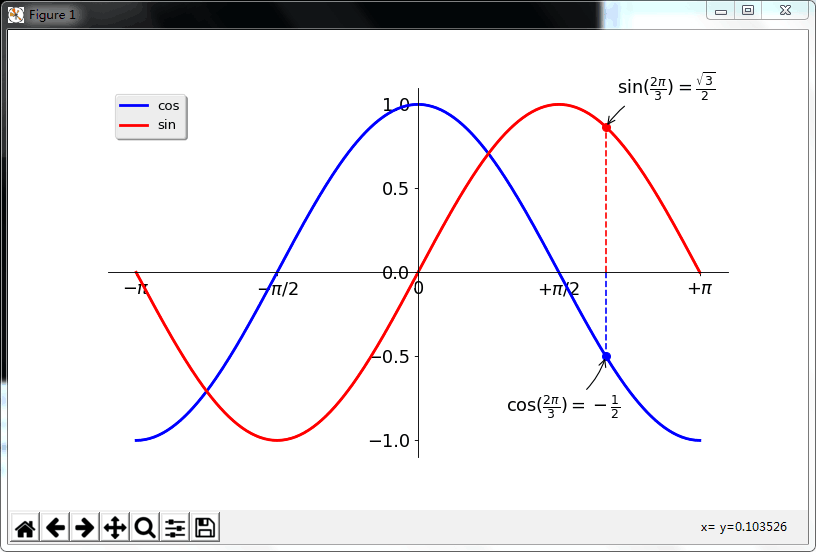微信文章：https://mp.weixin.qq.com/s/njYYVivJos4EgmWD7J7KHw

iPython Notebook HTML：http://ooou6mjma.bkt.clouddn.com/sincos.html


展开全文python
• 这是之前做的一个在直角坐标系数学函数得小程序。 首先我的讲一下画笔的画图规则： 例： 我们开始建立一个画布时他的坐标原点在左上角，为了方便我我们正常的画图 我们的重新设置坐标原点 代码如下： pDC-...
这是之前做的一个在直角坐标系中画数学函数得小程序。
首先我的讲一下画笔的画图规则：
例：
我们开始建立一个画布时他的坐标原点在左上角，为了方便我我们正常的画图
我们的重新设置坐标原点
代码如下：
pDC-> SetViewportOrg(point/2,point/2);//设置中心坐标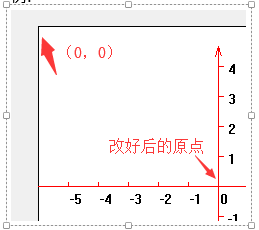MoveTo(x,y);//起点
LineTo(x,y);//连线的点
TextOut(x,y,a);//a为所显示的内容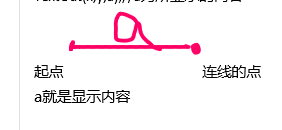而这个程序的主要思想其实就是不断得在界面上画图。图中得网格，刻度
都是用画笔画的：
网格：
CPen *pPenblue = new CPen(); //创建画笔对象
pPenblue ->CreatePen(PS_SOLID, 1, RGB(0, 0, 255)); //蓝色画笔
pDC ->SelectObject(pPenblue);
for(int z=0;z<=point/10;z++)
{
pDC ->MoveTo(-point/2,-point/2+10*z);
pDC ->LineTo(point/2,-point/2+10*z);
pDC ->MoveTo(-point/2+10*z,-point/2);
pDC ->LineTo(-point/2+10*z,point/2);
}
point的其实就是控制画布大小的，用来放大和缩小，刻度的位置确定等，贯穿整个程序。
画函数图像：
先确定起点第一个起点，再连接到第一个终点，在确定第二个起点，连接到第二个终点，其实第二个的起点就是第一个的终点，依次类推，for（）其实就是用来确定范围的
代码如下：
//确定画笔起点
for(x=-point/2*100;x<=point/2*100;x++)
{
m=x*0.01;
y=(atof(a)*pow(m,4)+atof(b)*pow(m,3)+atof(c)*pow(m,2)+atof(d)*m+atof(e))*(point/20);//表达式
y=y+(u-1)*(point/20)-f*(point/20);
m=m*(point/20)+(point/20)*(r-1)-(point/20)*(l-1);
if(y<point/2&&y>-point/2&&m>-(point/2)&&m<(point/2))//规定画图范围
{
pDC ->MoveTo(m,y);
break;
}
}
for(int x=-point/2*100;x<=point/2*100;x++)//确定连线的点
{
m=x*0.01;
y=(atof(a)*pow(m,4)+atof(b)*pow(m,3)+atof(c)*pow(m,2)+atof(d)*m+atof(e))*(point/20);//表达式
y=y+(u-1)*(point/20)-f*(point/20);
m=m*(point/20)+(point/20)*(r-1)-(point/20)*(l-1);
if(y<point/2&&y>-point/2&&m>-(point/2)&&m<(point/2))//规定画图范围
{
pDC ->LineTo(m,y); //竖起轴
}
}
改程序中还实现了上下左右放大和缩小的功能：
放大缩小：
就像我刚刚所说的一样，通过调节point值得大小，来放大和缩小所画图像得大小。
上下左右：
通过定义几个变量，放比例调节几个变量得大小
u=1,f=1,l=1,r=1;
U是用来向上移动用的
f是向下移动用的
l向左移动用的
r是向右移动用的
例向下移动：
向下和向左得数值是负的，所以f,l的值要减去。
没次按下向下的键，所以f++;f的值就增大。
代码如下：
for(x=-point/2*100;x<=point/2*100;x++)
{
m=x*0.01;
y=(atof(a)*pow(m,4)+atof(b)*pow(m,3)+atof(c)*pow(m,2)+atof(d)*m+atof(e))*(point/20);//表达式
y=y+(u-1)*(point/20)-f*(point/20);
m=m*(point/20)+(point/20)*(r-1)-(point/20)*(l-1);
if(y<point/2&&y>-point/2&&m>-(point/2)&&m<(point/2))//规定画图范围
{
pDC ->MoveTo(m,y);
break;
}
}
for(int x=-point/2*100;x<=point/2*100;x++)//确定连线的点
{
m=x*0.01;
y=(atof(a)*pow(m,4)+atof(b)*pow(m,3)+atof(c)*pow(m,2)+atof(d)*m+atof(e))*(point/20);//表达式
y=y+(u-1)*(point/20)-f*(point/20);
m=m*(point/20)+(point/20)*(r-1)-(point/20)*(l-1);
if(y<point/2&&y>-point/2&&m>-(point/2)&&m<(point/2))//规定画图范围
{
pDC ->LineTo(m,y); //竖起轴
}
}
f++;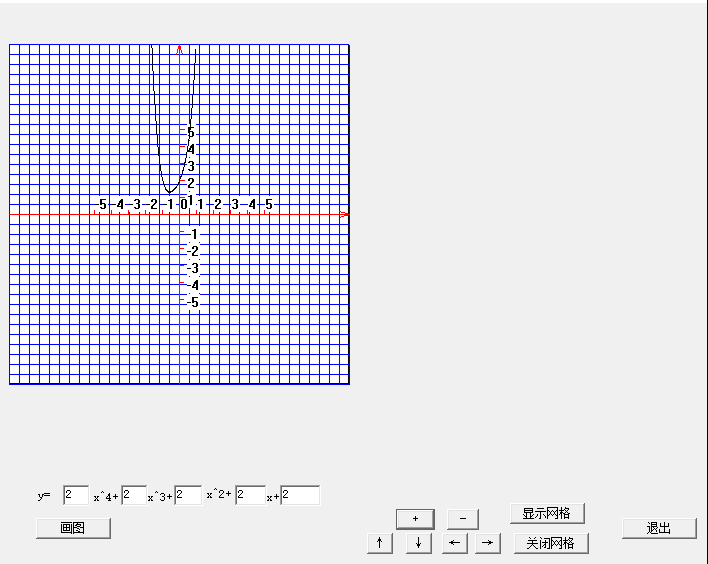希望对大家有帮助！！！
源代码下载：https://download.csdn.net/download/qq_38977566/10794602
展开全文C MFC
• 世界坐标系是任意选定的，可以任意事先定义，然后给出每个特征点在世界坐标系下的三维坐标，然后以一定顺序存储这些点。 特征点的像素坐标，一般是通过角点检测算法直接得到的，角点检测算法有他自己的存储点的顺序...
一、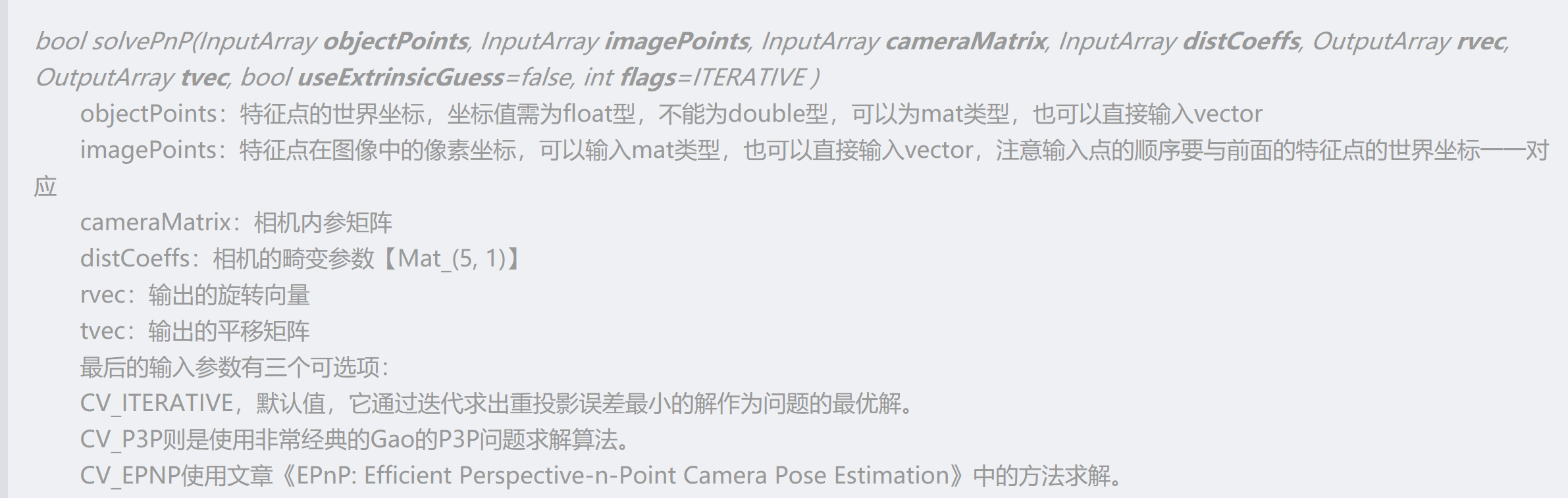二、
世界坐标系是任意选定的，可以任意事先定义，然后给出每个特征点在世界坐标系下的三维坐标，然后以一定顺序存储这些点。
特征点的像素坐标，一般是通过角点检测算法直接得到的，角点检测算法有他自己的存储点的顺序，此时需要把输入的像素点的存储顺序人为改变，使其和输入的世界坐标的顺序对应相同。
角点检测算法有他自己的存储点的顺序，或者不改变像素点的存储顺序，然后根据点与点的对应关系 确定世界坐标系。
三、旋转向量转换旋转矩阵
应用罗德里格斯变换
double rm;
cv::Mat rotM(3, 3, CV_64FC1, rm);
Rodrigues(rvec, rotM);
四、由旋转矩阵求得姿态角
代码：
float theta_z = atan2(rotMat, rotMat)*57.2958;
float theta_y = atan2(-rotMat, sqrt(rotMat * rotMat + rotMat * rotMat))*57.2958;
float theta_x = atan2(rotMat, rotMat)*57.2958;
============================================================================================= =============================================================================================
在OpenCV中，可以使用calibrateCamera函数，通过多个视角的2D/3D对应，求解出该相机的内参数和每一个视角的外参数。
使用C++接口时的输入参数如下：
objectPoints - 每一个视角中，关键点的世界坐标系。可以使用vector < vector <Point3f> >类型，第一层vector表示每一个视角，第二层vector表示每一个点。如果使用OpenCV自带的棋盘格，可以直接传入交叉点（不包括边角）的实际坐标，以物理世界尺度（例如毫米）为单位。写坐标时，要保证z轴为0，按照先x变化，后y变化，从小到大的顺序来写。如果网格尺寸为5厘米，写作：(0,0,0),(5,0,0), (10,0,0)...(0,5,0), (5,5,0), (10,5,0),...如下图例子，x方向是8个交叉点，y方向3个较差点。
imagePoints - 每一个视角中，关键点的图像坐标系。可以使用vector < vector <Point2f> >类型。
这个值可以通过findChessboardCorners函数从图像中获得。注意：传入findChessboardCorners函数的patternSize参数，要和objectPoints中的行列数统一。
imageSize - 图像尺寸。
flags - 参数。决定是否使用初始值，扭曲参数个数等。
输出参数如下：
cameraMatrix - 3*3的摄像机内矩阵。
distCoeffs - 4*1（具体尺寸取决于flags）。对图像坐标系进行进一步扭曲。
这两个参数是内参数，可以把摄像机坐标系转换成图像坐标系。
rvecs - 每一个视图的旋转向量。vector<Mat>类型，每个vec为3*1，可以用Rodrigues函数转换为3*3的旋转矩阵。
tvecs - 每一个视图的平移向量。vector<Mat>类型，每个vec为3*1。
这两个参数是外参数，每一个视图不同，可以把世界坐标系转换成摄像机坐标系。

问题：这个世界坐标系是什么样的？
在objectPoints中，我们实际只设定了每个交叉点的间隔，具体xyz指向哪里却没有给出。
这时候，需要使用drawChessboardCorners函数，把检测到的2D点在原图上显示出来：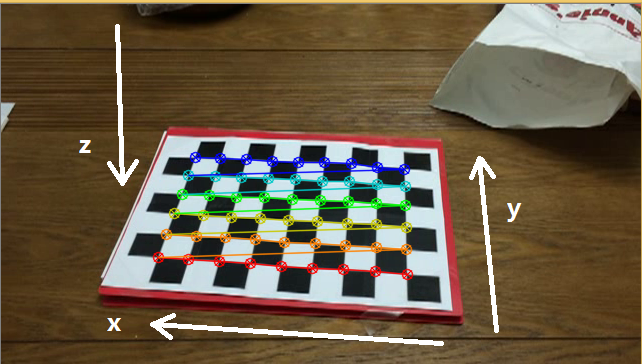这个函数按照先变化x，而后变化y的方式依次画点。先画的行为红色，逐渐变为蓝色。
回顾我们写objectPoints时的方式，可以推断出世界坐标的方向：图中x轴正方向向左，y轴正方向远离镜头，根据右手螺旋，z轴正方向向下。
总结来说，calibrateCamera函数给出的世界坐标方向，是由obejctPoints的设定顺序，以及findChessboardCorners的检测顺序共同决定的。
但是，findChessboardCorners先检测那个角点并不确定。前例从右下角开始，以下就从左上角开始：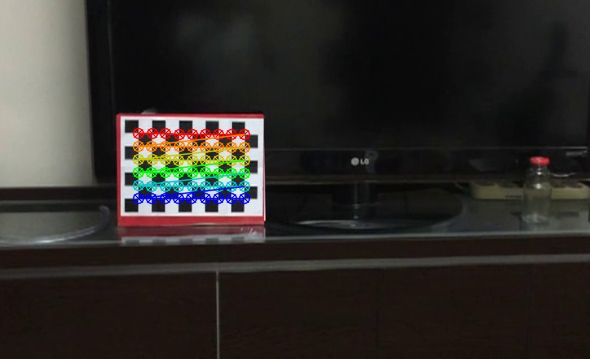另一种常见用法是：先用calibrateCamera标定好摄像机内参，而后使用solvePnP函数只求解外参。solvePnP函数的参数意义和calibrateCamera类似。 ---------------------  作者：shenxiaolu1984  来源：CSDN  原文：https://blog.csdn.net/shenxiaolu1984/article/details/50165635 
展开全文• 坐标函数 import matplotlib.pyplot as plt import numpy as np #设置[-3,3]区间的横轴，分成50份 x=np.linspace(-3,3,50) y=2*x+1 #坐标轴 plt.plot(x,y) plt.show() 这是核心框架，之后的操作都是围绕...
这是我们要做的最终效果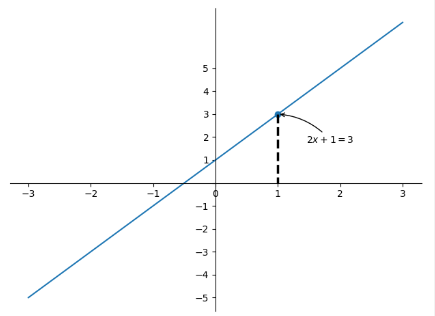接下来将分为3步
画出坐标与函数
import matplotlib.pyplot as plt
import numpy as np
#设置[-3,3]区间的横轴，分成50份
x=np.linspace(-3,3,50)
y=2*x+1
#画出坐标轴
plt.plot(x,y)
plt.show()

这是核心框架，之后的操作都是围绕着修改plt进行的，效果如下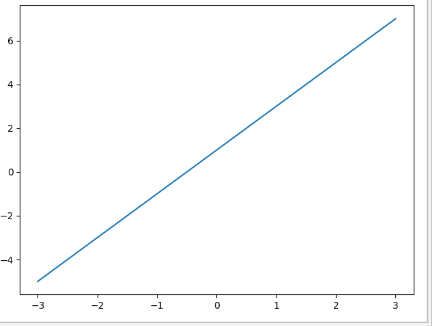修改坐标原点
在plt.show()上面加上
#将y轴分成[-5,5]的11份
new_ticks=np.linspace(-5,5,11)
plt.yticks(new_ticks)

# gca表示get current axes获得当前实例对象，个人觉得有点像java中的this
ax=plt.gca()
#隐藏右边和上边的边框
ax.spines['right'].set_color('none')
ax.spines['top'].set_color('none')

#ax获得x轴，并将x轴与y中的0对齐
ax.xaxis.set_ticks_position('bottom')
ax.spines['bottom'].set_position(('data',0))
#ax获得y轴，并将y轴与x中的0对齐
ax.yaxis.set_ticks_position('left')
ax.spines['left'].set_position(('data',0))

#隐藏掉y轴的0坐标，不然和x轴重了，不好看，0位于从下到上第6个
yticks = ax.yaxis.get_major_ticks()
yticks.label1.set_visible(False)

效果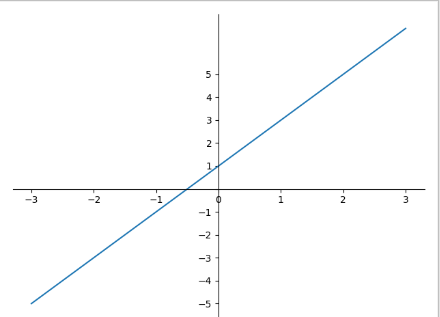添加annotation注解
在刚刚的代码下方加上
#在坐标系中标记处一个点
x0=1
y0=2*x0+1
plt.scatter(x0,y0)
#从点往下做垂线，从[x0,y0]到[x0,0],颜色是black 格式是--,宽度为2.5

plt.plot([x0,x0],[y0,0],'k--',lw=2.5)
plt.annotate(
r'$2x+1=%s$'%y0,xy=(x0,y0),#写上的字符串，字符串位置
xytext=(+30, -30),textcoords='offset points',#字符串相对偏移，指向的点
# 指向的样式，弧度
)

就完成了我们的最终效果
上面的r’$2x+1=%s$'是使用用了正则表达式，通过正则表达式有很完整的体系，我们可以设置很多好看的字体的实用的功能
当然了，matplotlib的能量远不止于此，继续加油学习鸭！
完整代码
#!/usr/bin/env python
# -*- coding: utf-8 -*-
# @Time    : 2019/2/15  23:34
# @Author  : cs
# @decription: 简单的坐标系与函数表达

import matplotlib.pyplot as plt
import numpy as np
x=np.linspace(-3,3,50)
y=2*x+1
plt.plot(x,y)

new_ticks=np.linspace(-5,5,11)
plt.yticks(new_ticks)

ax=plt.gca()
ax.spines['right'].set_color('none')
ax.spines['top'].set_color('none')
ax.xaxis.set_ticks_position('bottom')
ax.spines['bottom'].set_position(('data',0))
ax.yaxis.set_ticks_position('left')
ax.spines['left'].set_position(('data',0))

#隐藏掉y轴的0坐标，不然和x轴重了，不好看，0位于从下到上第6个
yticks = ax.yaxis.get_major_ticks()
yticks.label1.set_visible(False)

#在坐标系中标记处一个点
x0=1
y0=2*x0+1
plt.scatter(x0,y0)
#从点往下做垂线，从[x0,y0]到[x0,0],颜色是black 格式是--,宽度为2.5
plt.plot([x0,x0],[y0,0],'k--',lw=2.5)
plt.annotate(
r'$2x+1=%s$'%y0,xy=(x0,y0),#写上的字符串，字符串位置
xytext=(+30, -30),textcoords='offset points',#字符串相对偏移，指向的点
# 指向的样式，弧度
)
plt.show()

展开全文数据可视化
• ## Visio画坐标系步骤

万次阅读 2020-03-09 22:04:18
1. 2. 3. 4. 5. 6.第五步的内容用来画函数的直线部分
• 本文使用MFC的CDC类绘制三维坐标系及球面函数。首先计算推导出三维坐标在二维平面显示的坐标变换方程（使用斜二测视图），使用球面的参数方程，然后定义图形缩放比例规模、坐标轴位移，变换坐标系和规模等，最后绘制...mfc
• 世界坐标系原点在视图左上角，本例子通过世界坐标转换，将坐标原点定位在视图中央，Y轴向上，X轴向右，并绘制坐标轴，基于逻辑坐标系下的绘图，可将转换关系函数取消生效，对比世界坐标系下的绘图。 重写...
• 本文使用MFC的CDC类绘制二维坐标系及正余弦函数，可以进行坐标变换、规模变换，可以设置绘制的函数。通过输入自变量的范围及步长，绘制出相应的函数图形。mfc
• 可以任意输入数学函数，生成函数图像。 初次使用时，强烈推荐输入H回车查看帮助。
• 1 先讲一个取消操作，有时选中某个操作完成后想进入下一步但是不知道如何取消操作 如下：取消按键操作 点击指针工具就好了 2 直角坐标系：   完成 ...
•opengl 图形 gluOrtho2D 计算机图形学
• %以下3行为坐标轴平行的直线，颜色为灰色 plot3([-4,4],[0,0],[0,0],'--','Color',[0.5 0.5 0.5]); hold on plot3([0,0],[-4,4],[0,0],'--','Color',[0.5 0.5 0.5]); plot3([0,0],[0,0],[-4,4],'--','Color',...matlab
• ## 球坐标系

万次阅读 多人点赞 2019-06-28 14:56:48
坐标系是三维坐标系的一种，用以确定三维空间中点、线、面以及体的位置，它以坐标原点为参考点，由方位角、仰角和距离构成。球坐标系在地理学、天文学中都有着广泛应用。 球坐标系 　球坐标中是这样表示空间中...
• MFC 画坐标系以及灰度图像的直方图分类： VC++2009-08-01 16:46 1350人阅读 评论(0) 收藏 举报http://blog.csdn.net/zhenxiaohui/article/details/44000591.创建一个对话框类CHistogramdmfc delete 文档 null 存储
• 1、平面直角坐标系； 二、程序运行结果 三、numpy.hstack()函数 1、函数    函数原型：numpy.hstack(tup)，其中tup是arrays序列，阵列必须具有相同的形状，除了对应于轴的维度（默认情况下，第一个）。    ...
• 直角坐标系（Rectangular coordinates）   在平面内画两条互相垂直，并且有公共原点的数轴。其中横轴为X轴，纵轴为Y轴。这样我们就说在平面上建立了平面直角坐标系，简称直角坐标系。  坐标系所在平面叫做坐标...极坐标
• 两个函数函数原型如下： glVertex(GLfloat x, GLfloat y）;...简单的说前者的两个参数所代表的坐标点，必须落在后者参数所形成的坐标系内，不然该点就在viewport之外。 举个例子： void dOpenGL 视图
• 坐标为每10s传感器采集的数据 代码：using System; using System.Collections; using System.Collections.Generic; using System.ComponentModel; using System.Data; using System.IO; using System.Drawing; ...vs2010 graphics c#曲线
• 其实弄懂坐标变换的关键是理解什么是“眼睛坐标系”(eye coordinate system) 和“目标坐标系”(object coordinate system)，以及各个API函数使用的是哪套坐标：是“眼睛坐标”还是“目标坐标”。  所谓“眼睛坐标...
• python绘制笛卡尔直角坐标系 有些数学题目经常要用到数形结合思想，尤其是一些函数，如果能够把函数图像出来进行解题的话，往往会更加清晰明了。matplotlib python
• 简单介绍arcgis软件中坐标系定义、设置和常见问题。
• 0.各种变换调用顺序和各种坐标系出现顺序 1.各种坐标 a.从绘图这个角度来看 世界坐标系和当前绘图坐标系 世界坐标： 世界坐标系以屏幕中心为原点(0, 0, 0)。你面对屏幕，你的右边是x正轴，上面是y正轴...
• x = 0:0.01:pi; f = 4*cos(x)+3*sin(x); g = 5*cos(x)+6*sin(x); plot(x,f,'r',x,g,'b');matlab
• 摄像机矩阵由内参矩阵和外参矩阵组成，对摄像机矩阵进行QR分解可以得到内参矩阵和...外参包括旋转矩阵R3×3、平移向量T3×1，它们共同描述了如何把点从世界坐标系转换到摄像机坐标系，旋转矩阵描述了世界坐标系的坐...
• ## MFC 绘图坐标系

千次阅读 2016-08-22 11:12:41
主要为三个坐标系：窗口坐标系、客户区坐标系、屏幕坐标系 窗口坐标系： 以对话框窗口左上角为坐标原点，包括窗口的标题栏、菜单栏、工具栏等。 客户区坐标系： 客户区坐标都必须有一个客户对象，是以客户对象...MFC...